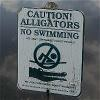68

0   3,548   1 day
NOW-HERE-NO-WHERE74

0   3,189   1 day
NOW-HERE-NO-WHERE58

0   2,826   1 day
NOW-HERE-NO-WHERE49

0   1,383   4 days
NOW-HERE-NO-WHERE73

0   2,626   3 weeks
NOW-HERE-NO-WHERE56

0   2,602   3 weeks
NOW-HERE-NO-WHERE74

0   2,637   3 weeks
NOW-HERE-NO-WHERE94

0   3,435   4 weeks
NOW-HERE-NO-WHERE50

0   2,597   6 weeks
Rick82

0   2,984   7 weeks
NOW-HERE-NO-WHERE82

0   2,417   7 weeks
NOW-HERE-NO-WHERE81

0   3,077   3 months
NOW-HERE-NO-WHERE98

0   2,903   3 months
NOW-HERE-NO-WHERE86

0   2,680   3 months
NOW-HERE-NO-WHERE66

0   2,745   3 months
NOW-HERE-NO-WHERE69

0   2,418   3 months
NOW-HERE-NO-WHERE59

0   2,336   3 months
NOW-HERE-NO-WHERE45

0   2,262   3 months
NOW-HERE-NO-WHERE58

0   2,301   3 months
NOW-HERE-NO-WHERE100

0   5,771   3 months
NOW-HERE-NO-WHERE-->